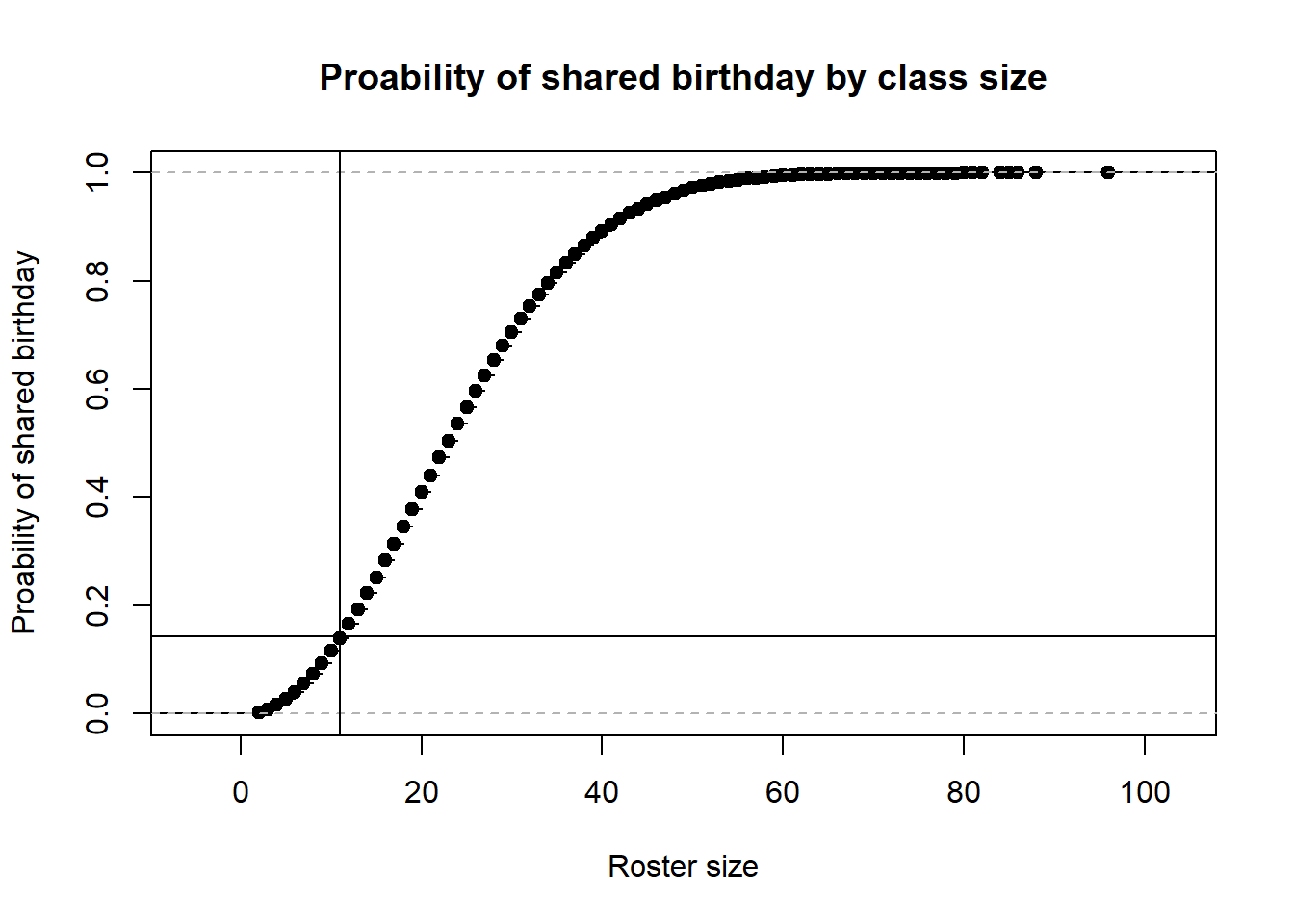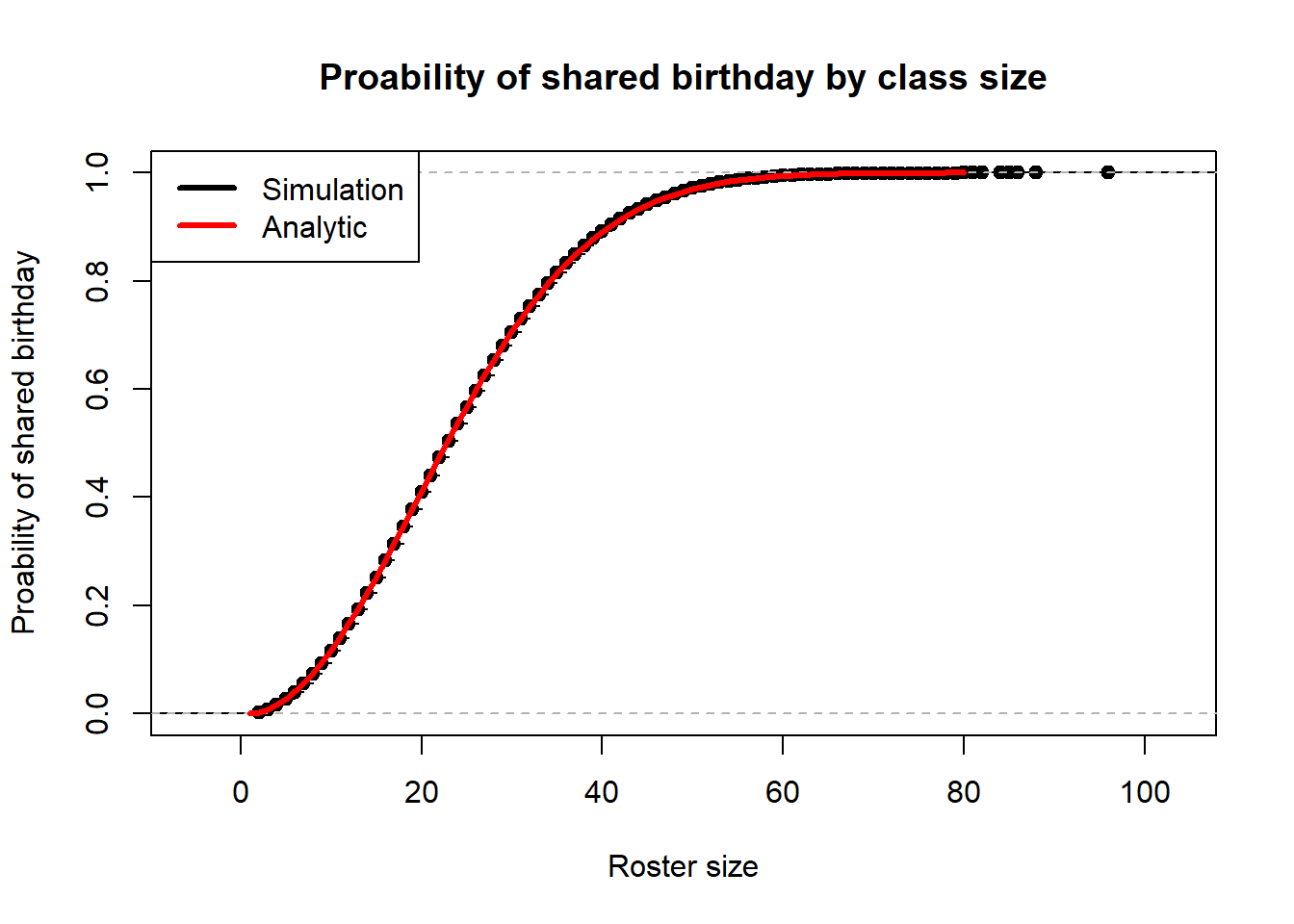## The puzzle

Suppose there is a class of size n. Explanation …

## Simulation solution

This is how we solved the BP with simulation.

set.seed(1)

The second thing we did was write a function to mimic roster creation.

one_roster <- function(S = 11){
birthdays <- sample(x = 365, size = S, replace = TRUE)
any(duplicated(birthdays))
}

R <- 100000

We used our function to create 10^{5} rosters.

out <- rep(NA, R)
for(i in 1:R){
out[i] <- one_roster()
}

out <- replicate(R, one_roster())

For a roster size of 11, we found the probability to be 0.14286.

## Additional Code
first_duplicate <- function(){
birthdays <- sample(x = 365, size = 366, replace = TRUE)
min(which(duplicated(birthdays)))
}

out3 <- replicate(R, first_duplicate())
#hist(out3, freq = FALSE)

plot(ecdf(out3), xlab = "Roster size", ylab = "Proability of shared birthday", main = "Proability of shared birthday by class size")
abline(h=mean(out), v = 11)## Analytic solution

We can use math notation in the commentary. $y = \alpha + \beta x + \epsilon$

$P(\text{shared birthday} | \text{roster size = n}) = 1-\frac{365 \cdot 364 \cdots (365 - n + 1)}{365^n} = 1 - \frac{{365 \choose n}n!}{365^n}$

# Analytic solution
plot(ecdf(out3), xlab = "Roster size", ylab = "Proability of shared birthday", main = "Proability of shared birthday by class size")
bp <- function(n) 1-exp(lchoose(365,n) + lfactorial(n) - n*log(365))
lines(1:80, bp(1:80), col = "red", lwd = 3)
legend("topleft", legend = c("Simulation","Analytic"), col = c("black","red"), lwd = 3)